# Combinator Birds

## Details

The data is imported from this table of Combinator Birds, which in turn is taken from "To Mock a Mockingbird" by Raymond Smullyan.
The default content is a Dataset containing the following properties for each named combinator:
 "Symbol" the symbol used to represent the combinator in the "Rule" property "Rule" the operation of the combinator expressed via a transformation Rule "Arguments" the number of arguments expected for the combinator to apply "SK Basis Form" the combinator written as an expression in the SK combinator basis

## Examples

### Basic Examples

Get the rules corresponding to each combinator bird:

 In:=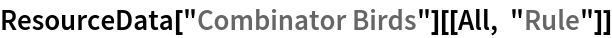Out=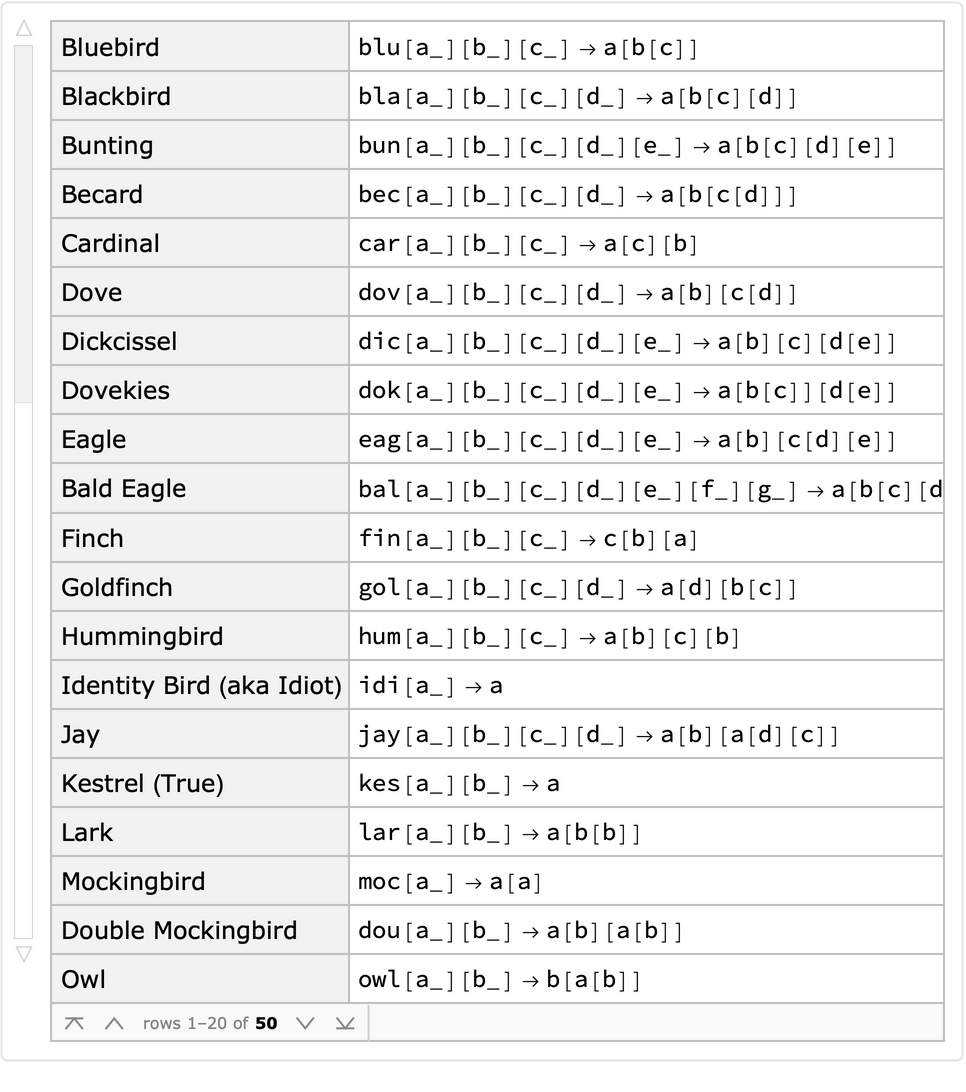Select combinators from the Dataset by their symbols:

 In:=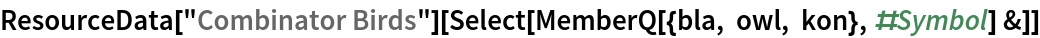Out=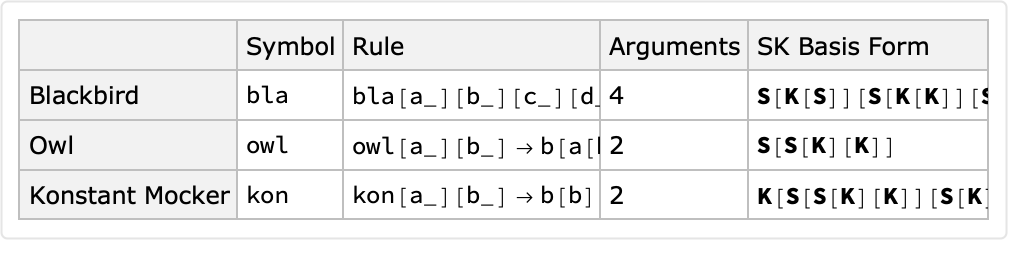Use combinator rules to transform a combinator expression to its fixed point, at which it can no longer evolve:

 In:=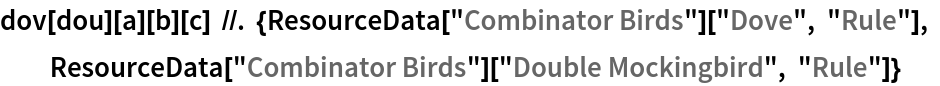Out=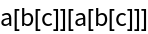Use combinator rules to evolve a combinator expression for a certain number of steps with a given traversal scheme:

 In:=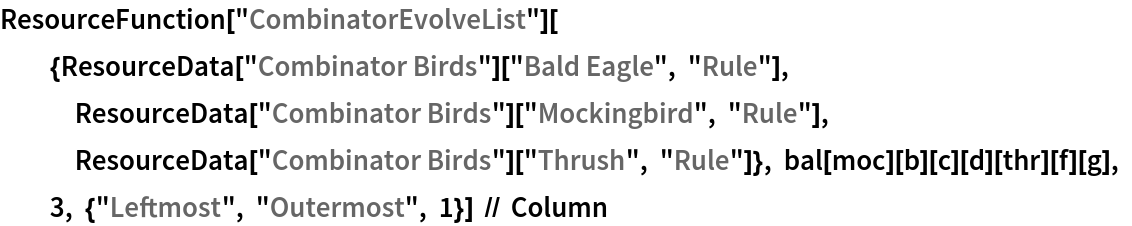Out=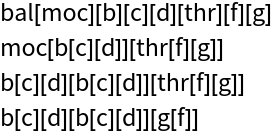In:=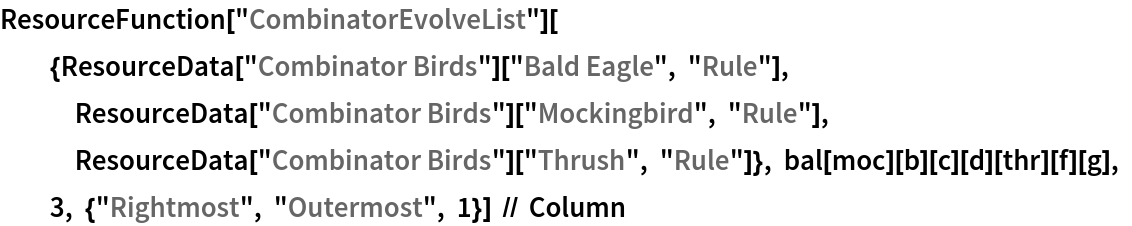Out=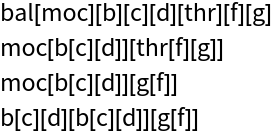Use the SK basis form of a combinator in its rule:

 In:=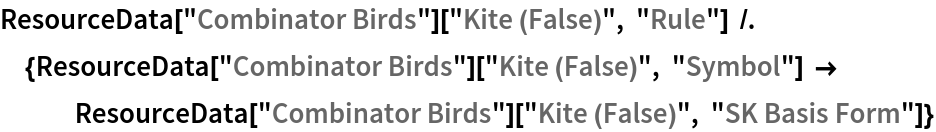Out=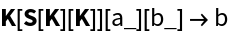### Visualizations

Visualize the possible updates at each step in the evolution of a combinator given certain rules:

 In:=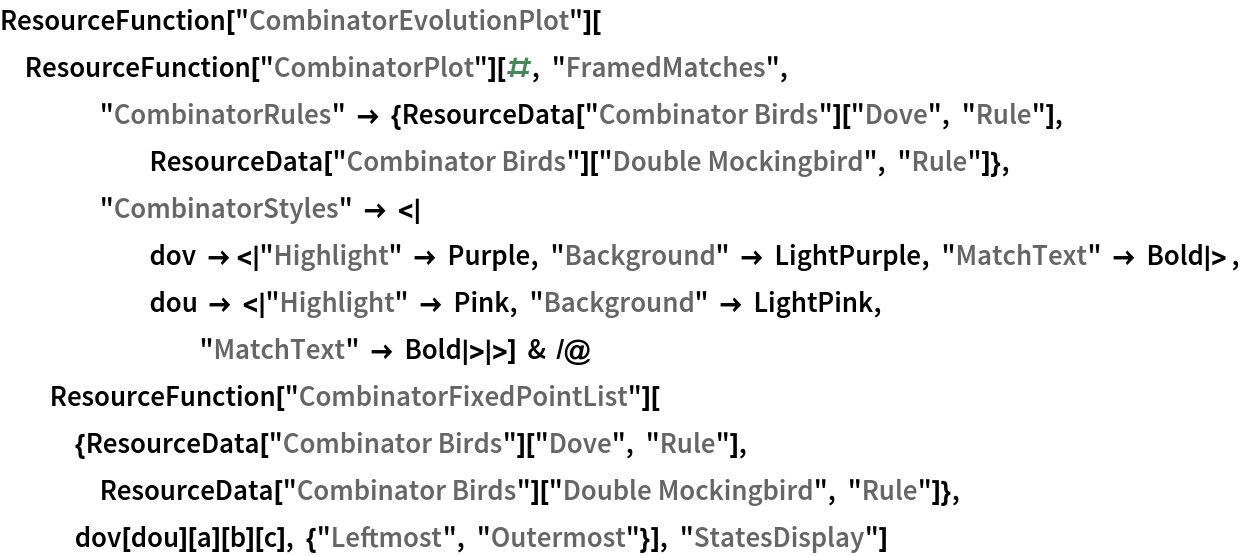Out=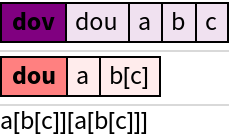Wolfram Research, "Combinator Birds" from the Wolfram Data Repository (2021)